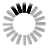# Hex Quiz

## For Programmers

A quiz to help memorize the 4-bit hex nibbles from 0 to F in binary. Why would you do this? Hexadecimal is the way to write binary in most modern programming languages and development tools. If you've ever done a bitwise operation on an integer or used a calculator to convert hex, this could save you some time. Converting hex to binary in your head may sound complicated but if you know the 16 nibbles (half-bytes) from 0 to F and their conversion to 0000-1111 you know it all.

Hint: first convert to decimal - e.g. E is the 5th letter of the alphabet, 5-1 is 4 so E = 14. C is the 3rd, so C = 12. Then count from 8, 4, 2, 1, setting the bits in that order to add up to the number. E.g. 10 is 8 + 2, so 1010.

Become one with your hex editor! Build your next CRUD app in x86 opcodes!

0x

In binary?